# Choquet simplex

(diff) ← Older revision | Latest revision (diff) | Newer revision → (diff)
A non-empty compact convex setin a locally convex space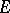that possesses the following property: Under the imbedding ofas the hyperplane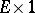in the space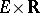the projecting cone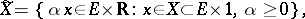oftransforms the spaceinto a partially ordered spacefor which the space generated by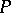, which is the space of differences, is a lattice. In the case whenis finite-dimensional, a Choquet simplex is an ordinary simplex with number of vertices equal to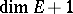. There exists a number of equivalent definitions of a Choquet simplex (see ). One of them reduces to the requirement that an intersection ofwith any translate of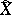should be again a translate of.
When, in addition to the above requirements,is separable andis metrizable, then forto be a Choquet simplex it is necessary and sufficient that any pointis the centre of gravity of the unique measure concentrated at the extreme points of. The concept of a Choquet simplex is essential when studying the uniqueness of an integral representation of a function (see , ). It was introduced by G. Choquet.